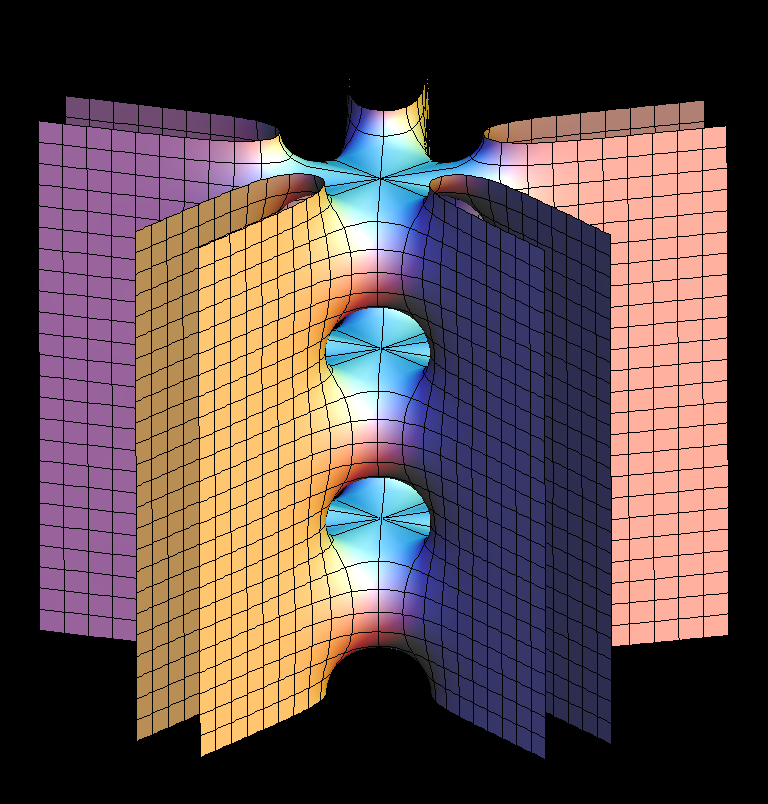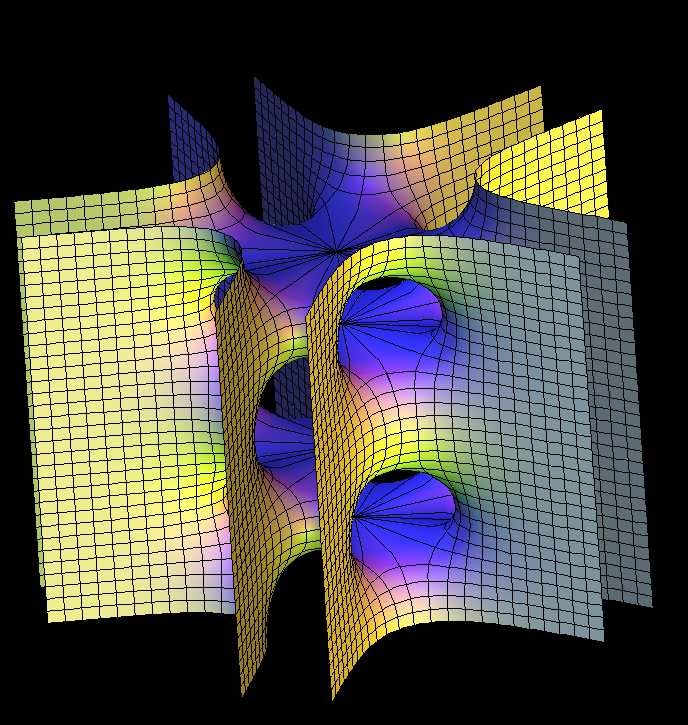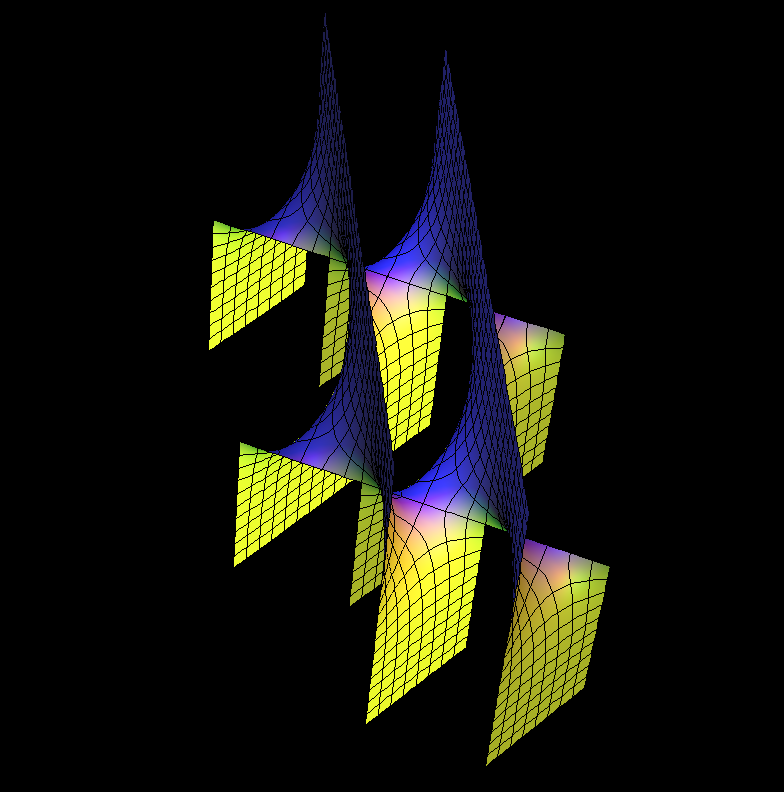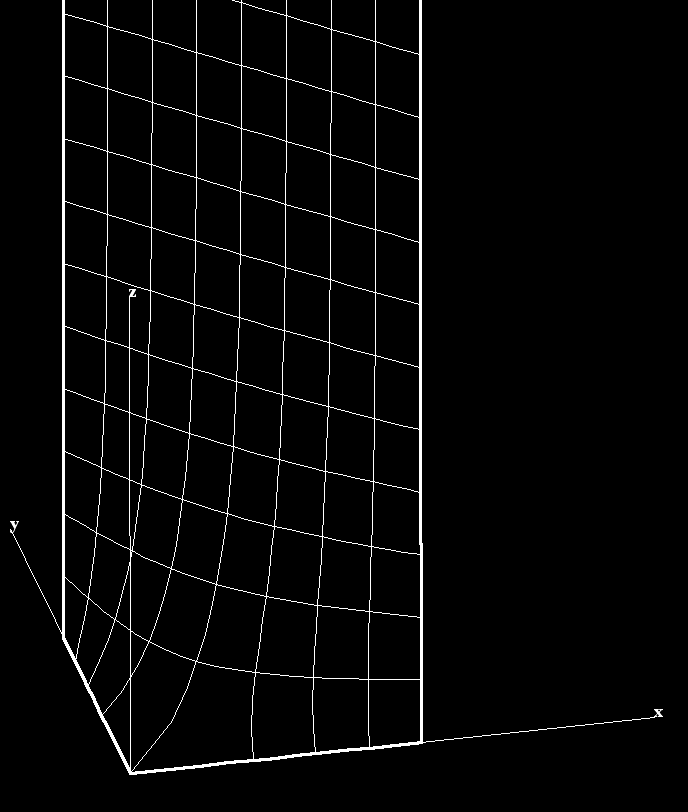Saddle Tower is Scherk's singly periodic minimal surface generalized to a family, by Hermann Karcher in 1988.

[see Scherk Surface]

## Dihedral Symmetry D5Scherk's singly periodic minimal surface generalized to a family and to dihedral symmetry of D5Scherk's singly periodic minimal surface generalized to a family and to dihedral symmetry of D5

[see Scherk Surface]

## Doubly PeriodicScherk's doubly periodic minimal surface generalized to a familyThis fundamental piece of the doubly periodic surface is bounded by two straight segments and two half lines. This boundary configuration spans a soap film equal to the shown minimal surface; It's a solution of the Plateau problem.

Plateau's problem is to show the existence of a minimal surface with a given boundary. The question was raised by Joseph-Louis Lagrange in 1760.

Physicist Joseph Plateau conducted extensive studies of soap films and formulated Plateau's laws which describe the structures formed by such films in foams. Plateau convinced mathematicians that Lagrange's problem probably has an answer.

For more, see Wikipedia Plateau's problem

These examples generalize Scherk's conjugate pair of singly-periodic/doubly-periodic minimal surfaces. [see Scherk Surface]

The singly-periodic examples stay embedded if the dihedral symmetry (and with it the number of punctures) is increased (Gauss(z)=z^k , k=ee-1). The most symmetric ones (bb=0.5/ee) can be deformed by decreasing bb. See [K1], [K2] for more details.

These surfaces are parametrized by punctured spheres, but the Weierstrass integrals have periods, a vertical one in the singly periodic case, two horizontal ones for doubly periodic surfaces. The parameter lines extend polar coordinates around the punctures to the whole sphere---in these cases giving level lines on the surfaces.

The degree of dihedral symmetry is, of course, a discrete property, and it is controlled by the parameter ee. Thus, ee should be set to an integer (the default is 2). For each choice of ee, changing bb gives a one-parameter family of surfaces, of which the most symmetric member is obtained by setting bb = 0.5/ee. Try setting ee to 3 and 4, and bb to 0.333 and 0.25 respectively. The wings of the singly periodic SaddleTower surfaces become *parallel in pairs* if (for ee>2 ) one sets bb = 0.0825. These stay embedded for ee=3 and ee=4.

• [K1] H. Karcher, Embedded minimal surfaces derived from Scherk's examples, Manuscripta Math. 62 (1988) pp. 83--114.
• [K2] Construction of Minimal Surfaces, H Karcher , in “Surveys in Geometry”, Univ. of Tokyo, 1989, and Lecture Notes No. 12, SFB 256, Bonn, 1989, pp. 1 — 96.

For a discussion of techniques for creating minimal surfaces with various qualitative features by appropriate choices of Weierstrass data, see either [KWH], or pages 192--217 of [DHKW].

• [KWH] H. Karcher, F. Wei, and D. Hoffman, The genus one helicoid, and the minimal surfaces that led to its discovery, in “Global Analysis in Modern Mathematics, A Symposium in Honor of Richard Palais' Sixtieth Birthday”, K. Uhlenbeck Editor, Publish or Perish Press, 1993
• [DHKW] U. Dierkes, S. Hildebrand, A. Kuster, and O. Wohlrab, Minimal Surfaces I, Grundlehren der math. Wiss. v. 295 Springer-Verlag, 1991

#### Related Surface

Get red/blue stereo glasses from amazon